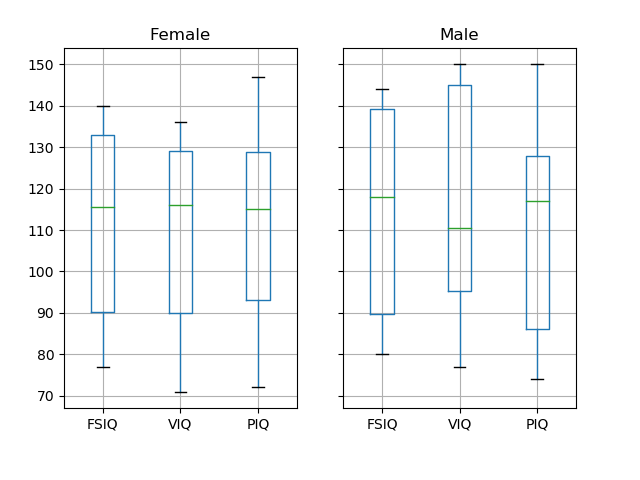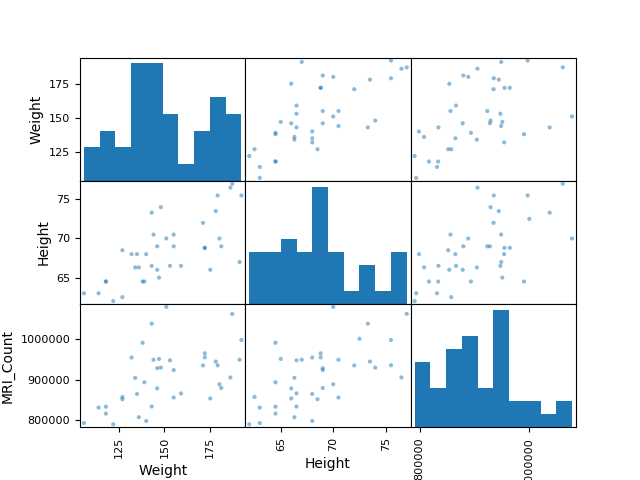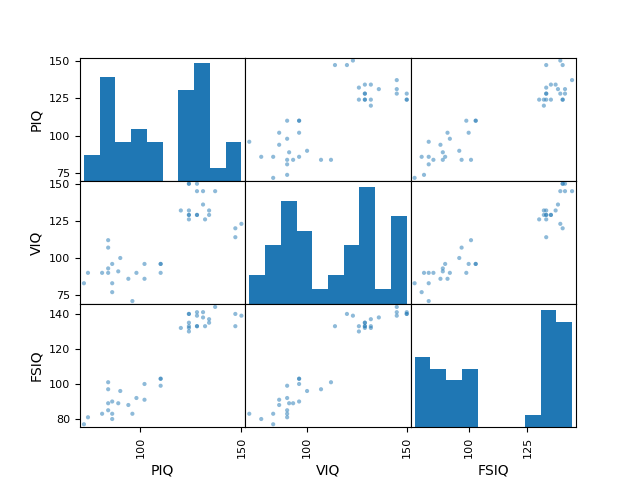# 3.1.6.2. Plotting simple quantities of a pandas dataframe¶

This example loads from a CSV file data with mixed numerical and categorical entries, and plots a few quantities, separately for females and males, thanks to the pandas integrated plotting tool (that uses matplotlib behind the scene).

•••```import pandas

# Box plots of different columns for each gender
groupby_gender = data.groupby('Gender')
groupby_gender.boxplot(column=['FSIQ', 'VIQ', 'PIQ'])

from pandas.tools import plotting

# Scatter matrices for different columns
plotting.scatter_matrix(data[['Weight', 'Height', 'MRI_Count']])
plotting.scatter_matrix(data[['PIQ', 'VIQ', 'FSIQ']])

import matplotlib.pyplot as plt
plt.show()
```

Total running time of the script: ( 0 minutes 0.597 seconds)

Gallery generated by Sphinx-Gallery